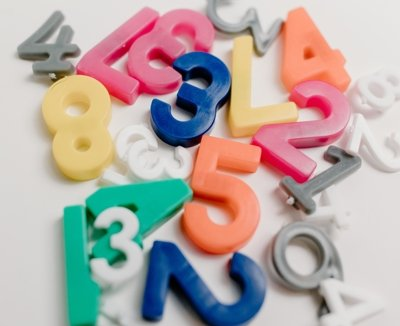Converting a binary number to a decimal number involves understanding the difference between the binary and decimal number systems, and then performing a series of calculations. The binary system is base 2, meaning it only uses the digits 0 and 1, while the decimal system is base 10 and uses the digits 0 through 9. To convert a binary number to decimal, you must assign a place value to each digit in the binary number, multiply each digit by its place value, and add up the results to obtain the decimal equivalent. With practice, converting binary to decimal becomes easier and can be done quickly and accurately.

This a exemple:

To convert a binary number to decimal, follow these steps:

1. Understand the difference between the binary and decimal number systems. Binary numbers are base 2 and only consist of the digits 0 and 1, while decimal numbers are base 10 and consist of the digits 0 through 9.
2. Write down the binary number you want to convert.
3. Determine the place value of each digit in the binary number. The rightmost digit has a place value of 1, the next digit to the left has a place value of 2, then 4, 8, 16, and so on.
4. Multiply each digit in the binary number by its place value and add up the results.
5. The sum you obtain in step 4 is the decimal equivalent of the binary number.

For example, to convert the binary number 10101 to decimal:

• The rightmost digit (1) has a place value of 1, the next digit (0) has a place value of 2, the next digit (1) has a place value of 4, the next digit (0) has a place value of 8, and the leftmost digit (1) has a place value of 16.
• Multiply each digit by its place value: 1×1 + 0x2 + 1×4 + 0x8 + 1×16 = 21.
• Therefore, the decimal equivalent of the binary number 10101 is 21.

This is example in javascript code:

``````function convertBinaryToDecimal(binary) {
let decimal = 0;
let baseValue = 0;

for(let i = binary.length - 1; i >= 0; i--) {
if(binary[i] === '1') decimal += Math.pow(2, baseValue);
baseValue++;
}
return decimal;
}``````

This is example in c# code:

``````static int ConvertBinaryToDecimal(string binaryNumber)
{
int decimalNumber = 0;
int baseValue = 1;

for (int i = binaryNumber.Length - 1; i >= 0; i--)
{
if (binaryNumber[i] == '1') decimalNumber += baseValue;
baseValue *= 2;
}
return decimalNumber;
}``````

Categories:

Tags:

## Comentários

Nenhum comentário para mostrar.